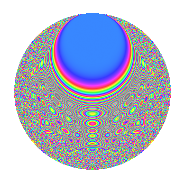Properties

 Label 6009.2.aLevel 6009 Weight 2 Character orbit a Rep. character $$\chi_{6009}(1,\cdot)$$ Character field $$\Q$$ Dimension 333 Newforms 4 Sturm bound 1336 Trace bound 2

Related objects

Defining parameters

 Level: $$N$$ = $$6009 = 3 \cdot 2003$$ Weight: $$k$$ = $$2$$ Character orbit: $$[\chi]$$ = 6009.a (trivial) Character field: $$\Q$$ Newforms: $$4$$ Sturm bound: $$1336$$ Trace bound: $$2$$

Dimensions

The following table gives the dimensions of various subspaces of $$M_{2}(\Gamma_0(6009))$$.

Total New Old
Modular forms 670 333 337
Cusp forms 667 333 334
Eisenstein series 3 0 3

The following table gives the dimensions of the cuspidal new subspaces with specified eigenvalues for the Atkin-Lehner operators and the Fricke involution.

$$3$$$$2003$$FrickeDim.
$$+$$$$+$$$$+$$$$74$$
$$+$$$$-$$$$-$$$$93$$
$$-$$$$+$$$$-$$$$92$$
$$-$$$$-$$$$+$$$$74$$
Plus space$$+$$$$148$$
Minus space$$-$$$$185$$

Trace form

 $$333q$$ $$\mathstrut +\mathstrut q^{2}$$ $$\mathstrut -\mathstrut q^{3}$$ $$\mathstrut +\mathstrut 335q^{4}$$ $$\mathstrut -\mathstrut 6q^{5}$$ $$\mathstrut +\mathstrut 3q^{6}$$ $$\mathstrut +\mathstrut 9q^{8}$$ $$\mathstrut +\mathstrut 333q^{9}$$ $$\mathstrut +\mathstrut O(q^{10})$$ $$333q$$ $$\mathstrut +\mathstrut q^{2}$$ $$\mathstrut -\mathstrut q^{3}$$ $$\mathstrut +\mathstrut 335q^{4}$$ $$\mathstrut -\mathstrut 6q^{5}$$ $$\mathstrut +\mathstrut 3q^{6}$$ $$\mathstrut +\mathstrut 9q^{8}$$ $$\mathstrut +\mathstrut 333q^{9}$$ $$\mathstrut +\mathstrut 2q^{10}$$ $$\mathstrut -\mathstrut 7q^{12}$$ $$\mathstrut -\mathstrut 6q^{13}$$ $$\mathstrut -\mathstrut 8q^{14}$$ $$\mathstrut +\mathstrut 6q^{15}$$ $$\mathstrut +\mathstrut 327q^{16}$$ $$\mathstrut -\mathstrut 10q^{17}$$ $$\mathstrut +\mathstrut q^{18}$$ $$\mathstrut -\mathstrut 4q^{19}$$ $$\mathstrut -\mathstrut 30q^{20}$$ $$\mathstrut -\mathstrut 4q^{21}$$ $$\mathstrut -\mathstrut 4q^{22}$$ $$\mathstrut +\mathstrut 15q^{24}$$ $$\mathstrut +\mathstrut 335q^{25}$$ $$\mathstrut -\mathstrut 22q^{26}$$ $$\mathstrut -\mathstrut q^{27}$$ $$\mathstrut +\mathstrut 28q^{28}$$ $$\mathstrut -\mathstrut 2q^{29}$$ $$\mathstrut +\mathstrut 6q^{30}$$ $$\mathstrut +\mathstrut 29q^{32}$$ $$\mathstrut +\mathstrut 12q^{33}$$ $$\mathstrut +\mathstrut 10q^{34}$$ $$\mathstrut -\mathstrut 4q^{35}$$ $$\mathstrut +\mathstrut 335q^{36}$$ $$\mathstrut +\mathstrut 18q^{37}$$ $$\mathstrut +\mathstrut 28q^{38}$$ $$\mathstrut -\mathstrut 14q^{39}$$ $$\mathstrut +\mathstrut 2q^{40}$$ $$\mathstrut -\mathstrut 14q^{41}$$ $$\mathstrut -\mathstrut 8q^{43}$$ $$\mathstrut +\mathstrut 28q^{44}$$ $$\mathstrut -\mathstrut 6q^{45}$$ $$\mathstrut +\mathstrut 20q^{46}$$ $$\mathstrut +\mathstrut 12q^{47}$$ $$\mathstrut +\mathstrut q^{48}$$ $$\mathstrut +\mathstrut 305q^{49}$$ $$\mathstrut +\mathstrut 11q^{50}$$ $$\mathstrut +\mathstrut 10q^{51}$$ $$\mathstrut +\mathstrut 18q^{52}$$ $$\mathstrut -\mathstrut 14q^{53}$$ $$\mathstrut +\mathstrut 3q^{54}$$ $$\mathstrut -\mathstrut 24q^{55}$$ $$\mathstrut -\mathstrut 4q^{56}$$ $$\mathstrut -\mathstrut 12q^{57}$$ $$\mathstrut -\mathstrut 10q^{58}$$ $$\mathstrut -\mathstrut 32q^{59}$$ $$\mathstrut -\mathstrut 2q^{60}$$ $$\mathstrut -\mathstrut 10q^{61}$$ $$\mathstrut -\mathstrut 8q^{62}$$ $$\mathstrut +\mathstrut 311q^{64}$$ $$\mathstrut -\mathstrut 32q^{65}$$ $$\mathstrut -\mathstrut 20q^{66}$$ $$\mathstrut -\mathstrut 8q^{67}$$ $$\mathstrut -\mathstrut 2q^{68}$$ $$\mathstrut +\mathstrut 20q^{69}$$ $$\mathstrut +\mathstrut 28q^{70}$$ $$\mathstrut -\mathstrut 20q^{71}$$ $$\mathstrut +\mathstrut 9q^{72}$$ $$\mathstrut -\mathstrut 22q^{73}$$ $$\mathstrut +\mathstrut 30q^{74}$$ $$\mathstrut -\mathstrut 23q^{75}$$ $$\mathstrut -\mathstrut 24q^{76}$$ $$\mathstrut -\mathstrut 44q^{77}$$ $$\mathstrut -\mathstrut 10q^{78}$$ $$\mathstrut +\mathstrut 12q^{79}$$ $$\mathstrut -\mathstrut 10q^{80}$$ $$\mathstrut +\mathstrut 333q^{81}$$ $$\mathstrut -\mathstrut 54q^{82}$$ $$\mathstrut -\mathstrut 48q^{83}$$ $$\mathstrut -\mathstrut 4q^{84}$$ $$\mathstrut -\mathstrut 20q^{85}$$ $$\mathstrut +\mathstrut 8q^{86}$$ $$\mathstrut +\mathstrut 14q^{87}$$ $$\mathstrut +\mathstrut 36q^{88}$$ $$\mathstrut -\mathstrut 66q^{89}$$ $$\mathstrut +\mathstrut 2q^{90}$$ $$\mathstrut -\mathstrut 52q^{91}$$ $$\mathstrut -\mathstrut 16q^{92}$$ $$\mathstrut +\mathstrut 4q^{93}$$ $$\mathstrut +\mathstrut 28q^{94}$$ $$\mathstrut +\mathstrut 4q^{95}$$ $$\mathstrut +\mathstrut 23q^{96}$$ $$\mathstrut -\mathstrut 14q^{97}$$ $$\mathstrut -\mathstrut 15q^{98}$$ $$\mathstrut +\mathstrut O(q^{100})$$

Decomposition of $$S_{2}^{\mathrm{new}}(\Gamma_0(6009))$$ into irreducible Hecke orbits

Label Dim. $$A$$ Field CM Traces A-L signs $q$-expansion
$$a_2$$ $$a_3$$ $$a_5$$ $$a_7$$ 3 2003
6009.2.a.a $$74$$ $$47.982$$ None $$-15$$ $$74$$ $$-34$$ $$-24$$ $$-$$ $$-$$
6009.2.a.b $$74$$ $$47.982$$ None $$-3$$ $$-74$$ $$14$$ $$-26$$ $$+$$ $$+$$
6009.2.a.c $$92$$ $$47.982$$ None $$17$$ $$92$$ $$34$$ $$22$$ $$-$$ $$+$$
6009.2.a.d $$93$$ $$47.982$$ None $$2$$ $$-93$$ $$-20$$ $$28$$ $$+$$ $$-$$

Decomposition of $$S_{2}^{\mathrm{old}}(\Gamma_0(6009))$$ into lower level spaces

$$S_{2}^{\mathrm{old}}(\Gamma_0(6009)) \cong$$ $$S_{2}^{\mathrm{new}}(\Gamma_0(2003))$$$$^{\oplus 2}$$